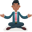Trusted answers to developer questions
Trusted Answers to Developer Questions

Related Tags

python

# What is seaborn.violinplot?Abhilash

Grokking Modern System Design Interview for Engineers & Managers

Ace your System Design Interview and take your career to the next level. Learn to handle the design of applications like Netflix, Quora, Facebook, Uber, and many more in a 45-min interview. Learn the RESHADED framework for architecting web-scale applications by determining requirements, constraints, and assumptions before diving into a step-by-step design process.### Overview

Seaborn is a data visualization library in Python that provides various functions for different charts and plots.

Refer Seaborn library in Python to learn more about seaborn.

A combination of the box and whisker plot is the same as the violin plot that displays the distribution of quantitative data over different levels of one or more categorical variables. The violin plot includes a kernel density estimation of the underlying distribution instead of a box plot, in which all plot elements correspond to real datapoints. This can be an efficient and appealing approach to display numerous data distributions at once.

### Syntax

seaborn.violinplot(x=None, y=None, hue=None, data=None, order=None, scale='area', gridsize=100, inner='box', orient=None, linewidth=None, color=None, palette=None)


### Parameters

• x, y, hue: These are inputs for plotting long-form data. These can be the names of the columns in the dataset.
• data: This is the dataset to be referred for the inputs x and y. If x and y are omitted, this will be taken as wide-form data for plotting.
• order: This is the order in which to plot the categorical levels in the dataset.
• scale: This indicates the method used to scale the width of each violin. This parameter can have the following values:
1. area: Every violin in the plot has the same area.
2. count: The width of the violins is proportional to the number of data points in the bin.
3. width: Every violin width will be the same.
• gridsize: This indicates the number of data points in the grid used to compute the kernel density estimate.
• inner: This parameter indicates the representation of the data points in the violin interior. This parameter can have the following values:
1. box: This draws a boxplot.
2. quartile: This draws the quartiles of the distribution.
3. point or stick: These show each underlying data point.
4. None: This draws unadorned violins.
• orient: This is the orientation of the plot, for example, v for vertical or h for horizontal.
• linewidth: This is the width of the gray lines on the plot frame.
• color: This is the color to use for all of the elements.
• palette: These are different colors to use for different levels.

### Example 1

import seaborn as snsgeyser = sns.load_dataset("geyser")v_plot = sns.violinplot(x="kind", y="duration", data=geyser)fig = v_plot.get_figure()fig.savefig("output/plot.png")
The violin plot

### Explanation

• Line 1: We import the seaborn library.
• Line 3: The seaborn library provides a predefined set of datasets. The different datasets can be found in seaborn datasets. One such dataset is the geyser dataset. The dataset is loaded into memory using the load_dataset() function.
• Line 5: A violin plot is created using the violinplot() function. Here, x is the kind of volcanic eruption, and y is the duration of the volcanic eruption.
• Lines 7 and 9: The plot is saved to the local disk as plot.png.

The plot above has inner as box (the default value). Hence, there’s a small boxplot in every violin.

### Example 2

import seaborn as snsgeyser = sns.load_dataset("geyser")v_plot = sns.violinplot(x="kind", y="duration", data=geyser, inner="stick")fig = v_plot.get_figure()fig.savefig("output/plot.png")
The violin plot

### Explanation

• Line 1: We import the seaborn library.
• Line 3: The seaborn library provides a predefined set of datasets. The different datasets can be found in seaborn datasets. One such dataset is the geyser dataset. The dataset is loaded into memory using the load_dataset() function.
• Line 5: A violin plot is created using the violinplot() function. Here, x is the kind of volcanic eruption, and y is the duration of the volcanic eruption.
• Lines 7 and 9: The plot is saved to the local disk as plot.png.

The plot above has inner as stick (the default value). Hence, the data points are indicated as horizontal lines in each violin.

RELATED TAGS

python

CONTRIBUTORAbhilash